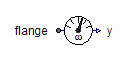SM Permanent Magnet Damper Cage - MapleSim Help

SM Permanent Magnet

Permanent magnet synchronous induction machineDescription

The SM Permanent Magnet component models a three phase permanent magnet synchronous induction machine. The resistance and stray inductance of the stator is modeled directly in stator phases, then using space phasor transformation and a rotor-fixed Air Gap model. The resistance and stray inductance of the rotor's squirrel cage is modeled in two axis of the rotor-fixed coordinate system. Permanent magnet excitation is modeled by a constant equivalent excitation current feeding the d-axis.

The following losses are modeled:

 • heat losses in the temperature dependent stator winding resistances
 • optional, when enabled: heat losses in the temperature dependent damper cage resistances
 • friction losses
 • core losses (only eddy current losses, no hysteresis losses)
 • permanent magnet losses

Whether a damper cage is present or not, can be selected with Boolean parameter useDamperCage (default = true).Connections

 Name Description Modelica ID $\mathrm{flange}$ Shaft flange ${i}_{}$ Damper cage currents ir ${\mathrm{idq}}_{\mathrm{dr}}$ Damper space phasor current / rotor fixed frame idq_dr ${\mathrm{plug}}_{\mathrm{sn}}$ Negative stator plug plug_sn ${\mathrm{plug}}_{\mathrm{sp}}$ Positive stator plug plug_sp $\mathrm{support}$ Support at which the reaction torque is acting support $\mathrm{thermalPort}$ thermalPortParametersGeneral Parameters

 Name Default Units Description Modelica ID ${f}_{s,\mathrm{nom}}$ 50 $\mathrm{Hz}$ Nominal frequency fsNominal ${J}_{r}$ 0.29 $\mathrm{kg}{m}^{2}$ Rotor moment of inertia Jr ${J}_{s}$ ${J}_{r}$ $\mathrm{kg}{m}^{2}$ Stator moment of inertia Js $p$ 2 Number of pole pairs (Integer) p ${T}_{r,\mathrm{oper}}$ 293.15 $K$ Operational temperature of (optional) damper cage TrOperational ${T}_{s,\mathrm{oper}}$ 293.15 $K$ Operational temperature of stator resistance TsOperational ${V}_{s,\mathrm{oc}}$ 293.15 $V$ Open circuit RMS voltage per phase at nominal frequency VsOpenCircuit Use Support Flange $\mathrm{false}$ True (checked) means stator support is enabled useSupport Use Thermal Port $\mathrm{false}$ True (checked) means heat port is enabled useThermalPortFriction Losses

 Name Default Units Description Modelica ID ${P}_{\mathrm{ref}}$ $0$ $W$ Reference friction losses PRef ${\omega }_{\mathrm{ref}}$ ${\omega }_{\mathrm{nom}}$ $\frac{\mathrm{rad}}{s}$ Reference angular velocity wRef ${p}_{\omega }$ $2$ $V$ Exponent of friction power_w

See Friction for details of the friction loss model.Permanent Magnet Losses

 Name Default Units Description Modelica ID $c$ $0$ Part of permanent magnet losses at zero current, independent of current c ${I}_{\mathrm{ref}}$ $A$ Reference stator RMS current IRef ${P}_{\mathrm{ref}}$ $0$ $W$ Reference permanent magnet losses at ${I}_{\mathrm{ref}}$ and ${\omega }_{\mathrm{ref}}$ PRef ${p}_{I}$ $2$ Exponent of permanent magnet loss torque w.r.t. stator current power_I ${p}_{\omega }$ $1$ Exponent of permanent magnet loss torque w.r.t. angular velocity power_w ${\omega }_{\mathrm{ref}}$ $\frac{\mathrm{rad}}{s}$ Reference angular velocity wRef

See Permanent Magnet Losses for details on the permanent magnet losses.Stator Core Losses

 Name Default Units Description Modelica ID ${P}_{\mathrm{ref}}$ $0$ $W$ Reference rotor core losses PRef ${V}_{\mathrm{ref}}$ $V$ Reference RMS voltage VRef ${\omega }_{\mathrm{ref}}$ $2\pi {f}_{s,\mathrm{nom}}$ $\frac{\mathrm{rad}}{s}$ Reference angular velocity wRef

See Core for details of the core loss model.Name Default Units Description Modelica ID ${I}_{\mathrm{ref}}$ ${I}_{{a}_{\mathrm{nom}}}$ $W$ Reference RMS current IRef ${P}_{\mathrm{ref}}$ $0$ $W$ Reference friction losses PRef ${\omega }_{\mathrm{ref}}$ ${\omega }_{\mathrm{nom}}$ $\frac{\mathrm{rad}}{s}$ Reference angular velocity wRef ${p}_{\omega }$ $1$ $V$ Exponent of stray load loss power_wName Default Units Description Modelica ID ${\mathrm{\alpha }}_{r}$ 0 $\frac{1}{K}$ Temperature coefficient of damper resistances in d- and q-axis alpha20r ${\mathrm{\alpha }}_{s}$ 0 $\frac{1}{K}$ Temperature coefficient of stator resistance at 20 degC alpha20s ${L}_{\mathrm{md}}$ $\frac{0.15\mathrm{\pi }}{{f}_{s,\mathrm{nom}}}$ $H$ Main field inductance in d-axis Lmd ${L}_{\mathrm{mq}}$ $\frac{0.15\mathrm{\pi }}{{f}_{s,\mathrm{nom}}}$ $H$ Main field inductance in q-axis Lmq ${L}_{r\sigma d}$ $\frac{0.025\mathrm{\pi }}{{f}_{s,\mathrm{nom}}}$ $H$ Damper stray inductance in d-axis Lrsigmad ${L}_{r\sigma q}$ ${L}_{r\sigma d}$ $H$ Damper stray inductance in q-axis Lrsigmaq ${L}_{s\sigma }$ $\frac{0.05\mathrm{\pi }}{{f}_{s,\mathrm{nom}}}$ $H$ Stator stray inductance per phase Lssigma ${L}_{\mathrm{s0}}$ ${L}_{s\sigma }$ $H$ Stator zero sequence inductance Lszero ${R}_{\mathrm{rd}}$ 0.04 $\mathrm{\Omega }$ Damper resistance in d-axis at TRef Rrd ${R}_{\mathrm{rq}}$ ${R}_{\mathrm{rd}}$ $\mathrm{\Omega }$ Damper resistance in q-axis at TRef Rrq ${R}_{s}$ 0.03 $\mathrm{\Omega }$ Warm damper resistance in q-axis Rs ${T}_{r,\mathrm{ref}}$ 293.15 $K$ Reference temperature of damper resistances in d- and q-axis TrRef ${T}_{s,\mathrm{ref}}$ 293.15 $K$ Reference temperature of stator resistance TsRef Use Damper Cage $\mathrm{false}$ True (checked) means damper cage is enabled useDamperCageModelica Standard Library The component described in this topic is from the Modelica Standard Library. To view the original documentation, which includes author and copyright information, click here.